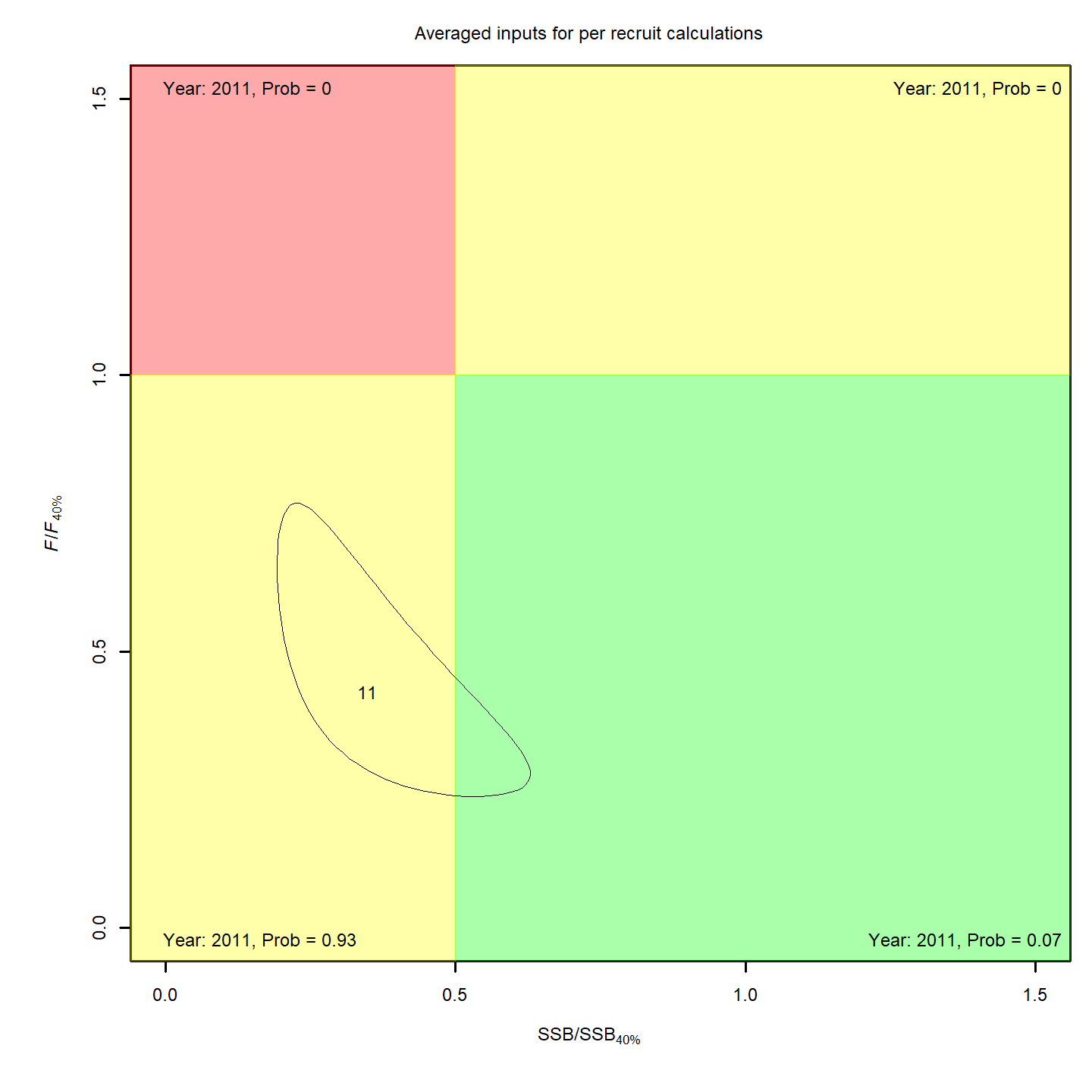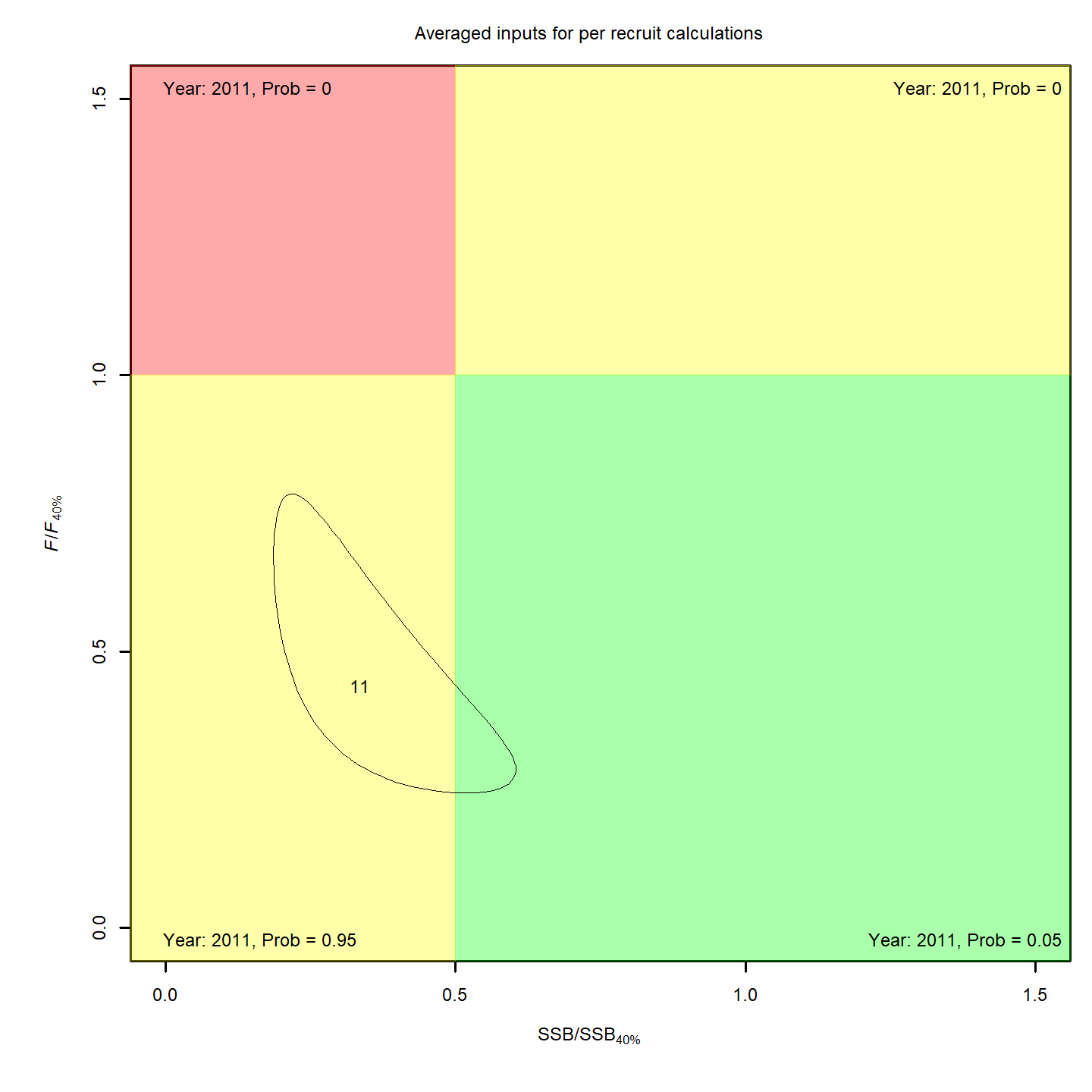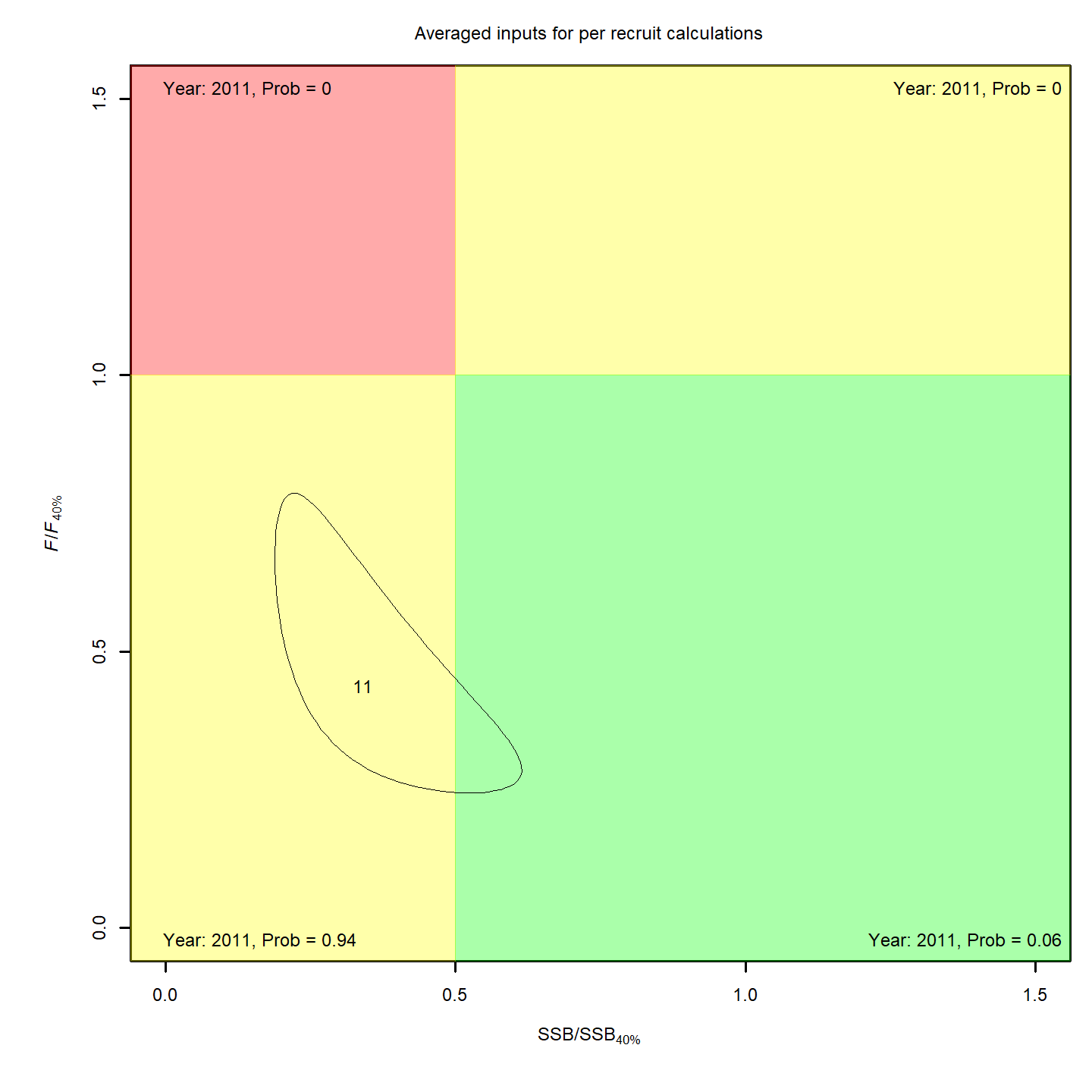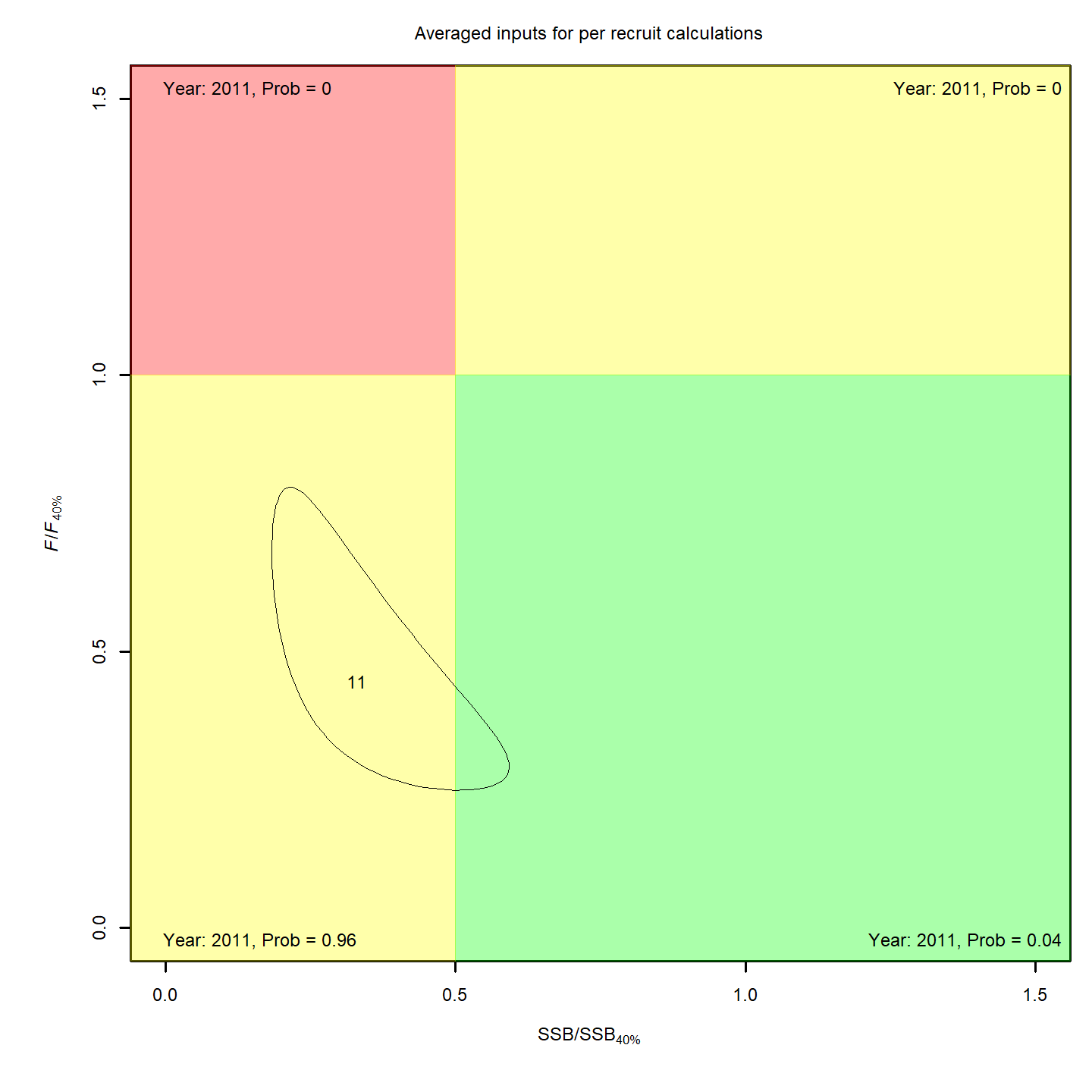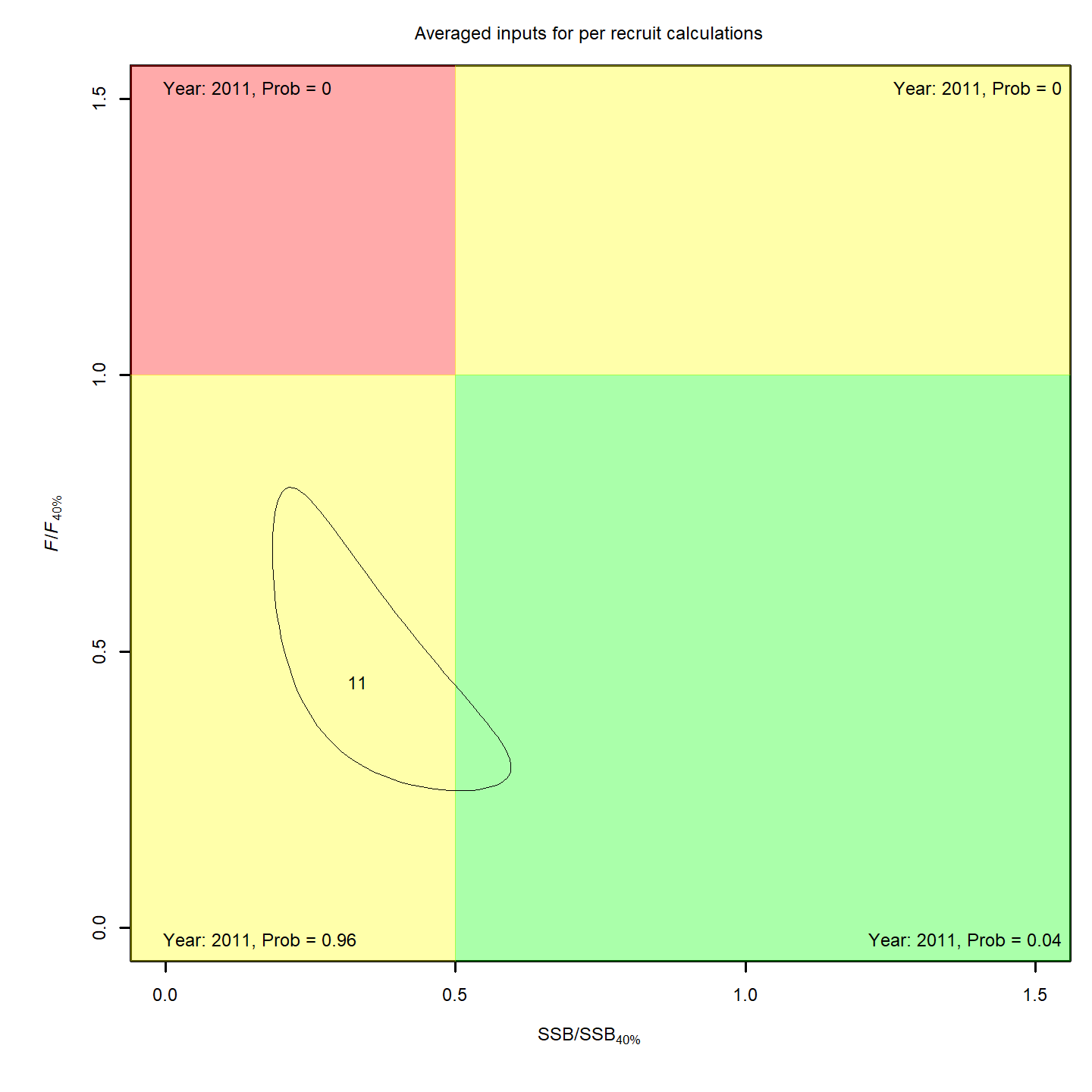In this vignette we walk through an example using the wham (WHAM = Woods Hole Assessment Model) package to run a state-space age-structured stock assessment model. WHAM is a generalization of code written for Miller et al. (2016) and Xu et al. (2018), and in this example we apply WHAM to the same stock, Southern New England / Mid-Atlantic Yellowtail Flounder.

This is the 2nd wham example, which builds off model m4 from example 1 (full state-space model, numbers at all ages are random effects, logistic normal age-compositions). We assume you already have wham installed. If not, see the Introduction. The simpler 1st example, without environmental effects, is available as a R script and vignette.

In example 2, we demonstrate how to specify and run WHAM with varying

• recruitment models (random, Bev-Holt, Ricker)

• environmental covariate (Cold Pool Index, CPI) process models (random walk, AR1), and

• how the CPI affects recruitment (controlling or limiting)

As in example 1, we check that each model converges (check_convergence()), plot diagnostics, results, and reference points (plot_wham_output()), and compare models using AIC and Mohn’s rho (compare_wham_models()).

## 1. Prepare wham

Open R and load the wham package:

library(wham)

For a clean, runnable .R script, look at ex2_CPI_recruitment_SNEMA_yellowtail.R in the example_scripts folder of the wham package install:

wham.dir <- find.package("wham")
file.path(wham.dir, "example_scripts")

You can run this entire example script with:

write.dir <- "choose/where/to/save/output" # otherwise will be saved in working directory
source(file.path(wham.dir, "example_scripts", "ex2_CPI_recruitment_SNEMA_yellowtail.R"))

Let’s create a directory for this analysis:

# choose a location to save output, otherwise will be saved in working directory
write.dir <- "choose/where/to/save/output"
dir.create(write.dir)
setwd(write.dir)

WHAM was built by modifying the ADMB-based ASAP model code (Legault and Restrepo 1999), and is designed to take an ASAP3 .dat file as input. We generally assume in wham that you have an existing ASAP3 .dat file. If you are not familiar with ASAP3 input files, see the ASAP documentation. For this vignette, an example ASAP3 input file is provided, ex2_SNEMAYT.dat. We will also need a data file with an environmental covariate, the Cold Pool Index, CPI.csv.

Copy ex2_SNEMAYT.dat and CPI.csv to our analysis directory:

wham.dir <- find.package("wham")
file.copy(from=file.path(wham.dir,"extdata","ex2_SNEMAYT.dat"), to=write.dir, overwrite=FALSE)
file.copy(from=file.path(wham.dir,"extdata","CPI.csv"), to=write.dir, overwrite=FALSE)

Confirm you are in the correct directory and it has the required data files:

list.files()
#>   "CPI.csv"                "ex1_SNEMAYT.dat"        "ex1_test_results.rds"
#>   "ex2_SNEMAYT.dat"        "ex2_test_results.rds"   "ex4_test_results.rds"
#>   "ex5_summerflounder.dat" "ex5_test_results.rds"   "ex6_test_results.rds"
#>  "GSI.csv"

Read the ASAP3 .dat file into R and convert to input list for wham:

asap3 <- read_asap3_dat("ex2_SNEMAYT.dat")

Load the environmental covariate (Cold Pool Index, CPI) data into R:

env.dat <- read.csv("CPI.csv", header=T)

We generally abbreviate ‘environmental covariate’ as ecov in the code. In this example, the ecov data file has columns for observations (CPI), standard error (CPI_sigma), and year (Year). Observations and year are always required. Standard error can be treated as fixed/data with yearly values (as here) or one overall value shared among years. It can also be estimated as a parameter(s), likewise either as yearly values or one overall value.

head(env.dat)
#>   Year     CPI CPI_sigma
#> 1 1973  0.5988    0.2838
#> 2 1974 -0.1760    0.2465
#> 3 1975 -1.1887    0.2539
#> 4 1976 -0.7938    0.2634
#> 5 1977 -0.6771    0.1576
#> 6 1978 -1.5195    0.2045

## 2. Specify models

Now we specify how the 7 models treat recruitment, the CPI process, and how the CPI affects recruitment:

df.mods <- data.frame(Recruitment = c(2,2,3,3,3,3,4),
ecov_process = c(rep("rw",4),rep("ar1",3)),
ecov_how = c(0,1,0,2,2,1,1), stringsAsFactors=FALSE)
n.mods <- dim(df.mods)
df.mods$Model <- paste0("m",1:n.mods) df.mods <- dplyr::select(df.mods, Model, tidyselect::everything()) # moves Model to first col Look at the model table df.mods #> Model Recruitment ecov_process ecov_how #> 1 m1 2 rw 0 #> 2 m2 2 rw 1 #> 3 m3 3 rw 0 #> 4 m4 3 rw 2 #> 5 m5 3 ar1 2 #> 6 m6 3 ar1 1 #> 7 m7 4 ar1 1 We specify the options for modeling recruitment and any environmental covariate(s) using the prepare_wham_input() function. WHAM provides 4 options for recruitment (recruit_model): 1. random walk, 2. random about mean, 3. Beverton-Holt, and 4. Ricker. The environmental covariate options are fed to prepare_wham_input() as a list, ecov:  m=1 # example for first model ecov <- list( label = "CPI", mean = as.matrix(env.dat$CPI),
logsigma = as.matrix(log(env.dat$CPI_sigma)), year = env.dat$Year,
use_obs = matrix(1, ncol=1, nrow=dim(env.dat)), # use all obs (all = 1)
lag = 1, # CPI in year t affects recruitment in year t+1
process_model = df.mods$ecov_process[m], # "rw" or "ar1" where = "recruit", # CPI affects recruitment how = df.mods$ecov_how[m]) # 0 = no effect, 1 = controlling, 2 = limiting

There are currently 2 options for the ecov process model (ecov$process_model): 1) random walk ('rw'), and 2) autoregressive ('ar1'). We must next specify where the ecov affects the population; here it is via recruitment (ecov$where = "recruit") as opposed to another process like catchability, mortality, maturity, etc. The options for how the ecov affects recruitment (ecov$how) follow Iles and Beverton (1998) and Xu et al. (2018): 1. “controlling” (dens-indep mortality), 2. “limiting” (carrying capacity, e.g. ecov determines amount of suitable habitat), 3. “lethal” (threshold, i.e. R –> 0 at some ecov value), 4. “masking” (metabolic/growth, ecov decreases dR/dS), and 5. “directive” (e.g. behavioral). Finally, we specify the lag at which CPI affects recruitment (ecov$lag = 1, i.e. CPI in year t affects recruitment in year t + 1).

You can set ecov = NULL to fit the model without environmental covariate data, but note that here we fit the ecov data even for models without an ecov effect on recruitment (m1 and m3) so that we can compare them via AIC (need to have the same data in the likelihood). We accomplish this by setting ecov$how = 0. Options are described in the prepare_wham_input() help page. Not all ecov$how options are implemented for every recruitment model.

?prepare_wham_input

## 3. Run the models

for(m in 1:n.mods){
# set up environmental covariate data and model options
ecov <- list(
label = "CPI",
mean = as.matrix(env.dat$CPI), logsigma = as.matrix(log(env.dat$CPI_sigma)),
year = env.dat$Year, use_obs = matrix(1, ncol=1, nrow=dim(env.dat)), # use all obs (=1) lag = 1, # CPI in year t affects recruitment in year t+1 process_model = df.mods$Ecov_process[m], # "rw" or "ar1"
where = "recruit", # CPI affects recruitment
how = df.mods$Ecov_how[m]) # 0 = no effect (but still fit Ecov to compare AIC) # (not used in this vignette) can set ecov = NULL to fit model without ecov data if(is.na(df.mods$ecov_process[m])) ecov = NULL

# generate wham input from ASAP3 and ecov data
input <- prepare_wham_input(asap3, recruit_model = df.mods$Recruitment[m], model_name = "Ex 2: SNEMA Yellowtail Flounder with CPI effects on R", ecov = ecov, NAA_re = list(sigma="rec+1", cor="iid")) # Builds off model m4 in example 1: # full state-space model: NAA_re = list(sigma="rec+1", cor="iid") # logistic normal age-compositions (below) # Age comp model = logistic normal pool obs (not multinomial, the default) input$data$age_comp_model_fleets = rep(5, input$data$n_fleets) # 5 = logistic normal (pool zero obs) input$data$n_age_comp_pars_fleets = c(0,1,1,3,1,2)[input$data$age_comp_model_fleets] input$data$age_comp_model_indices = rep(5, input$data$n_indices) # 5 = logistic normal (pool zero obs) input$data$n_age_comp_pars_indices = c(0,1,1,3,1,2)[input$data$age_comp_model_indices] n_catch_acomp_pars = c(0,1,1,3,1,2)[input$data$age_comp_model_fleets[which(apply(input$data$use_catch_paa,2,sum)>0)]] n_index_acomp_pars = c(0,1,1,3,1,2)[input$data$age_comp_model_indices[which(apply(input$data$use_index_paa,2,sum)>0)]] input$par$catch_paa_pars = rep(0, sum(n_catch_acomp_pars)) input$par$index_paa_pars = rep(0, sum(n_index_acomp_pars)) # Selectivity = logistic, not age-specific as in ex1 # 2 pars per block instead of n.ages # sel pars of indices 4/5 fixed at 1.5, 0.1 (specified via neg phase in ex2_SNEMAYT.dat) input$par$logit_selpars[1:4,7:8] <- 0 # last 2 rows will not be estimated (mapped to NA) # Fit model mod <- fit_wham(input, do.retro=TRUE, do.osa=TRUE) # Save model saveRDS(mod, file=paste0(df.mods$Model[m],".rds"))

# Plot output in new subfolder
plot_wham_output(mod=mod, dir.main=file.path(getwd(),df.mods$Model[m]), out.type='html') } ## 4. Check for convergence Collect all models into a list. mod.list <- paste0(df.mods$Model,".rds")
mods <- lapply(mod.list, readRDS)

There is no indication that any of the models failed to converge. In addition, SE estimates are calculable for all models (invertible Hessian, TMB::sdreport() succeeds).

vign2_conv <- lapply(mods, function(x) capture.output(check_convergence(x)))
for(m in 1:n.mods) cat(paste0("Model ",m,":"), vign2_conv[[m]], "", sep='\n')
#> Model 1:
#> stats:nlminb thinks the model has converged: mod$opt$convergence == 0
#> TMB:sdreport() was performed successfully for this model
#>
#> Model 2:
#> stats:nlminb thinks the model has converged: mod$opt$convergence == 0
#> TMB:sdreport() was performed successfully for this model
#>
#> Model 3:
#> stats:nlminb thinks the model has converged: mod$opt$convergence == 0
#> TMB:sdreport() was performed successfully for this model
#>
#> Model 4:
#> stats:nlminb thinks the model has converged: mod$opt$convergence == 0
#> TMB:sdreport() was performed successfully for this model
#>
#> Model 5:
#> stats:nlminb thinks the model has converged: mod$opt$convergence == 0
#> TMB:sdreport() was performed successfully for this model
#>
#> Model 6:
#> stats:nlminb thinks the model has converged: mod$opt$convergence == 0
#> TMB:sdreport() was performed successfully for this model
#>
#> Model 7:
#> stats:nlminb thinks the model has converged: mod$opt$convergence == 0
#> TMB:sdreport() was performed successfully for this model

## 5. Compare models

Calculate AIC and Mohn’s rho using compare_wham_models().

df.aic <- compare_wham_models(mods, sort=FALSE)$tab # can't sort yet bc going to make labels prettier df.mods <- cbind(df.mods, df.aic) Print and save the results table. m6 has the lowest AIC (Bev-Holt recruitment, CPI modeled as AR1, controlling effect of CPI on recruitment). # make results table prettier rownames(df.mods) <- NULL df.mods$Recruitment <- dplyr::recode(df.mods$Recruitment, 2='Random', 3='Bev-Holt', 4='Ricker') df.mods$ecov_how <- dplyr::recode(df.mods$ecov_how, 0='---',1='Controlling', 2='Limiting', 4='Masking') df.mods$NLL <- sapply(mods, function(x) round(x$opt$objective,3))
df.mods <- df.mods[order(df.mods$dAIC),] save("df.mods", file="vign2_res.RData") df.mods #> Model Recruitment Ecov_process Ecov_how NLL dAIC AIC rho_R #> m6 m6 Bev-Holt ar1 Controlling -824.636 0.0 -1509.3 0.2084 #> m7 m7 Ricker ar1 Controlling -824.196 0.9 -1508.4 0.2058 #> m5 m5 Bev-Holt ar1 Limiting -824.098 1.1 -1508.2 0.2159 #> m4 m4 Bev-Holt rw Limiting -813.377 20.5 -1488.8 0.2157 #> m2 m2 Random rw Controlling -810.097 25.1 -1484.2 0.2310 #> m3 m3 Bev-Holt rw --- -808.046 29.2 -1480.1 0.2331 #> m1 m1 Random rw --- -803.139 37.0 -1472.3 0.2592 #> rho_SSB rho_Fbar runtime #> m6 0.1037 -0.1154 1.6 #> m7 0.1002 -0.1124 1.7 #> m5 0.1087 -0.1197 1.7 #> m4 0.1091 -0.1201 1.7 #> m2 0.1151 -0.1274 1.4 #> m3 0.1060 -0.1167 1.5 #> m1 0.1124 -0.1264 1.3 ## 6. Results There are 3 options for plotting WHAM output. The default (out.type='html') creates and opens an HTML file with plots organized into tabs (code modified from r4ss::SS_html()): # save output plots in subfolder for each model for(m in 1:n.mods) plot_wham_output(mod=mods[[m]], dir.main=file.path(getwd(), df.mods$Model[m]), out.type='html')

### Cold Pool Index (CPI)

Models that included an effect of the Cold Pool Index on recruitment were strongly supported by AIC over models without CPI effects (m2 and m4-7 lower AIC than m1 and m3). Note that we can compare models with and without a CPI effect on recruitment using AIC because we also fit the CPI data in the models without the effect (m1 and m3).

Comparing m4 and m5 demonstrates that the CPI was best modeled as an AR1 process (m5) instead of a random walk (m4), since this was the only difference between the two models and m5 had lower AIC. In addition, the one-step-ahead residuals for the CPI from m5 (right) are smaller and conform more closely to a normal distribution than in m4 (left):Compared to the base model (m1, left), the best model that included CPI and SSB effects on recruitment, (m6, right) reduced the retrospective pattern in recruitment, $$\rho_R$$, from 0.259 to 0.208.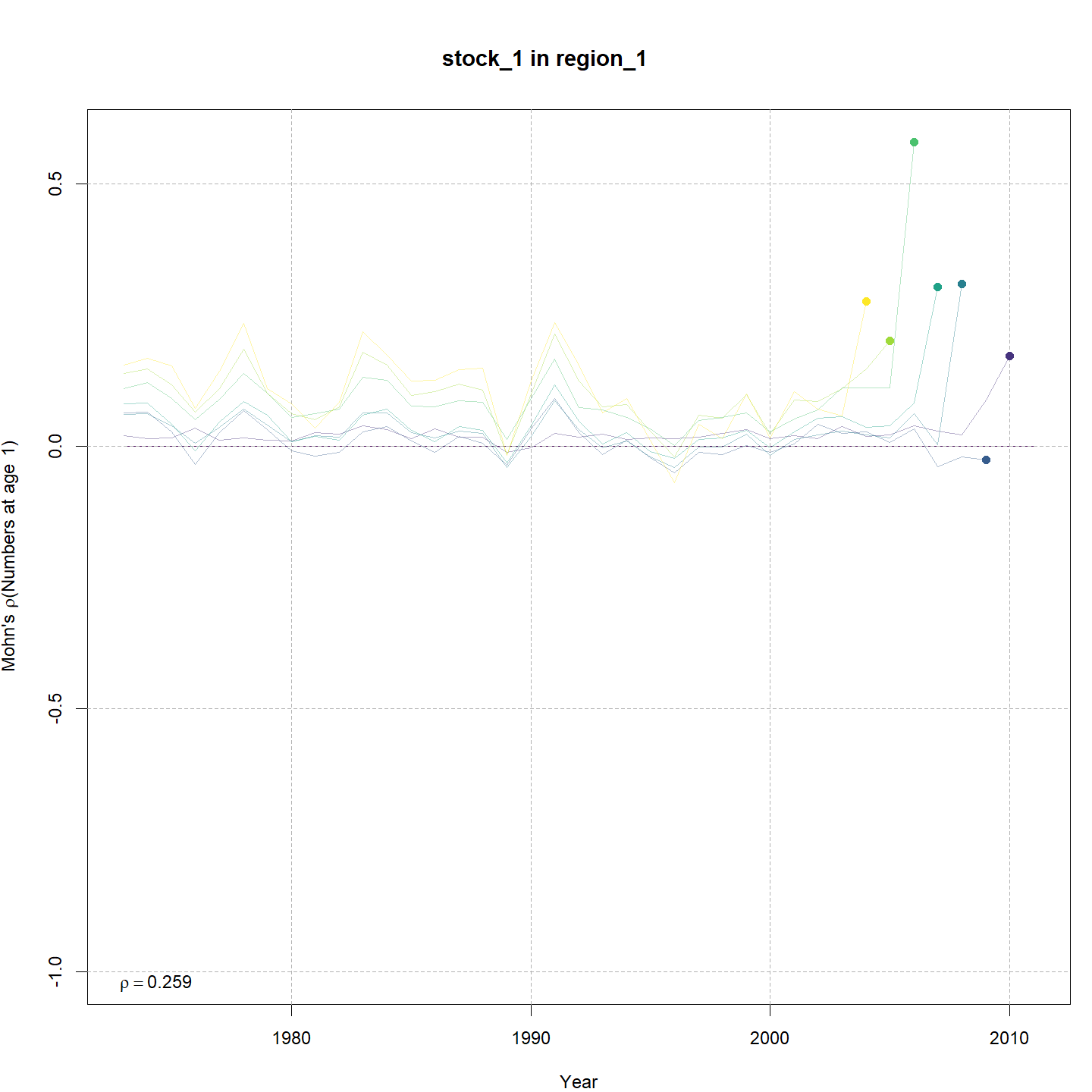### Recruitment

Beverton-Holt recruitment was strongly preferred over random (m4 lower AIC than m2) and weakly preferred over Ricker (m6 lower AIC than m7). Models that included both Bev-Holt and CPI effects on recruitment had lower AIC than the model with Bev-Holt but without the CPI (m4 vs. m3). Adding the CPI effect to the Bev-Holt explains some of the variability around the stock-recruit curve, which resulted in m4 (right) estimating lower $$\sigma^2_R$$ than m3 (left).There was weak evidence that the Cold Pool controls recruitment, as opposed to limiting recruitment (m5 vs. m6). The hypothesis that the CPI indicates the amount of suitable habitat for juvenile yellowtail flounder corresponds to a limiting effect, as in m5. In contrast, a controlling effect (as in m6) causes density-independent mortality. For more explanation, see Iles & Beverton (1998).

### Stock status

Whether or not to include the CPI had a greater influence on estimated stock status than whether, or how, to include a stock-recruit function. Specifically, the models differed in their estimation of the probability that the stock was overfished, $$Pr[SSB < 0.5 \: SSB_{40\%}]$$. Models that did not include an effect of the CPI on recruitment estimated higher probability that the stock was overfished (top row: m1 = 0.11 and m3 = 0.45; bottom row: m2 = 0.01, m4 = 0.02, and m6 = 0.04). All models estimated with 100% probability that the stock was not experiencing overfishing in 2011, $$F < F_{40\%}$$.